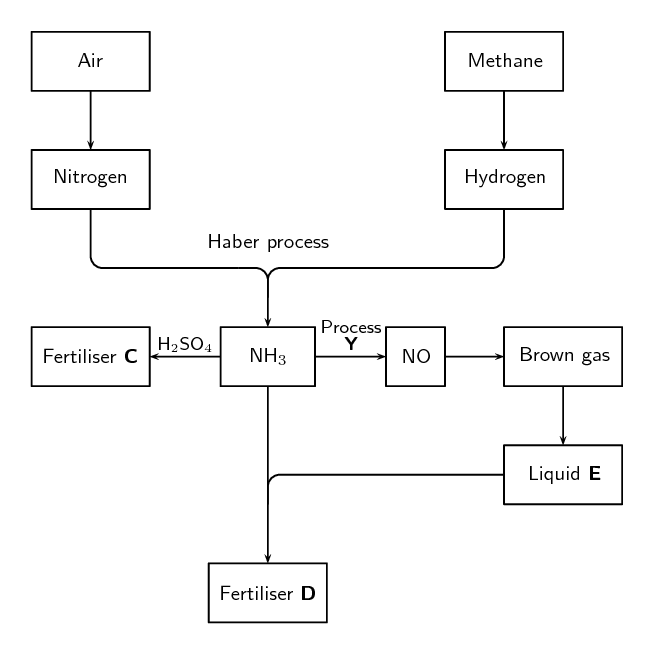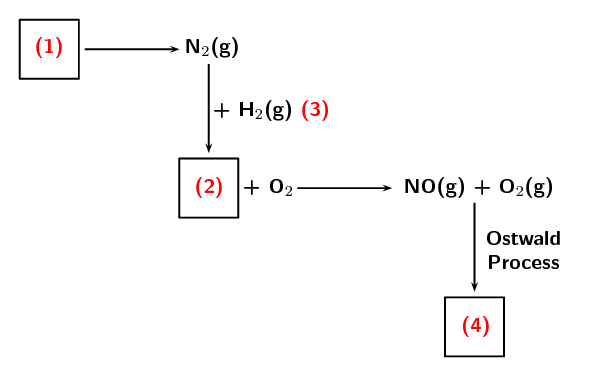We think you are located in United States. Is this correct?

# End of chapter exercises

Textbook Exercise 14.3

The following extract comes from an article on fertilisers:

A world without food for its people and a world with an environment poisoned through the actions of man are two contributing factors towards a disaster scenario.

Do you agree with this statement? Write down at least three arguments to substantiate your answer.

Learners give their own opinion.

There is likely to be a gap between food production and demand in several parts of the world by 2020. Demand is influenced by population growth and urbanisation, as well as income levels and changes in dietary preferences. While there is an increasing world population to feed, most soils in the world used for large-scale, intensive production of crops lack the necessary nutrients for the crops. This is where fertilisers can play a role.

The flow diagram below shows the main steps in the industrial preparation of two important solid fertilisers.How can fertilisers play a role in increasing the soil’s ability to produce crops?

Fertilisers supply nutrients to the soil in a soluble form which plants can then absorb.

Are the processes illustrated by the flow diagram above that of organic or inorganic fertilisers? Give a reason for your answer.

Inorganic fertilisers. Industrial processes are involved in the formation of these fertilisers, and they did not come from natural sources or living organisms.

Write down the balanced chemical equation for the formation of the brown gas.

$$2\text{NO}(\text{g}) + \text{O}_{2}(\text{g})$$ $$\rightleftharpoons$$ $$2\text{NO}_{2}(\text{g})$$

Write down the name of process Y.

Ostwald process

Write down the chemical formula of liquid E.

$$\text{HNO}_{3}$$

Write down the chemical formulae of fertilisers C and D respectively.

C - $$(\text{NH}_{4})_{2}\text{SO}_{4}$$

D - $$\text{NH}_{4}\text{NO}_{3}$$

The production of nitric acid is very important in the manufacture of fertilisers. Look at the diagram below, which shows part of the fertiliser production process, and then answer the questions that follow.Name the process at (1).

Fractional distillation of air

Name the gas at (2).

Ammonia

Name the process at (3) that produces gas (2).

Haber process

Name the immediate product at (4).

nitrogen dioxide

Name the final product of the Ostwald process (product (4) + $$\text{H}_{2}\text{O}$$).

nitric acid

Name two fertilisers that can be produced from nitric acid.

Nitrophosphates or ammonium nitrate.